# MP Board Class 6th Maths Solutions Chapter 14 Practical Geometry Ex 14.6

## MP Board Class 6th Maths Solutions Chapter 14 Practical Geometry Ex 14.6

Question 1.
Draw ∠POQ of measure 75° and find its line of symmetry.
Solution:
Steps of construction :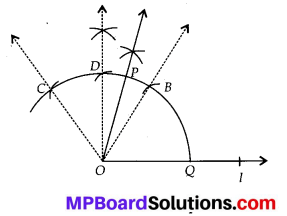(i) Draw a line l and mark a point O on it.
(ii) Place the pointer of the compasses at O and draw an arc of any radius which intersects the line l at Q.
(iii) Taking same radius, with centre Q, draw an arc which cuts the previous arc at B.
(iv) Join OB, then ∠BOQ = 60°.
(v) Taking same radius, with centre B, draw an arc which cuts the arc drawn in step
(ii) at C.
(vi) Draw bisector of ∠BOC which cuts the $$\widehat{B C}$$ at D. Thus, ∠DOQ = 90°.
(vii) Draw OP as bisector of ∠DOB. Thus, ∠POQ = 75°.

Question 2.
Draw an angle of measure 147° and construct its bisector.
Solution:
Steps of construction :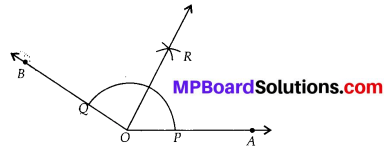(i) Draw OA.
(ii) With the help of protractor, draw ∠AOB = 147°.
(iii) Taking centre O and any convenient radius, draw an arc which intersects $$\overline{O A}$$ and $$\overline{O B}$$ at P and Q respectively.
(iv) Taking P as centre and radius more than half of PQ, draw an arc.
(v) Taking Q as centre and with the same radius, draw another arc which intersects the previous arc at R.
(vi) Join OR and produce it.
Thus, $$\overline{O R}$$ is the required bisector of ∠AOB.

Question 3.
Draw a right angle and construct its bisector.
Solution:
Steps of construction :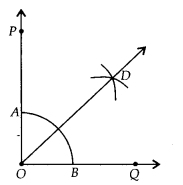(i) Draw $$\overline{O Q}$$.
(ii) With the help of protractor draw ∠QOP = 90°
(iii) Taking O as centre and any convenient radius, draw an arc which intersect the $$\overline{O P}$$ and $$\overline{O Q}$$ at A and B respectively.
(iv) Taking B and A as centre and radius more than half of BA, draw two arcs which intersect each other at the point D.
(v) Join OD. Thus, $$\overline{O D}$$ is the required bisector of ∠QOP.Question 4.
Draw an angle of measure 153° and divide it into four equal parts.
Solution:
Steps of construction :(i) Draw $$\overline{O A}$$.
(ii) At O, with the help of a protractor, draw ∠AOB = 153°.
(iii) Draw $$\overline{O C}$$ as the bisector of ∠AOB.
(iv) Again, draw $$\overline{O D}$$ as the bisector of ∠AOC.
(v) Again, draw $$\overline{O E}$$ as the bisector of ∠BOC.
(vi) Thus; $$\overline{O C}$$, $$\overline{O D}$$ and $$\overline{O E}$$ divide ∠AOB in four equal parts.

Question 5.
Construct with ruler and compasses, angles of following measures:
(a) 60°
(b) 30°
(c) 90°
(d) 120°
(e) 45°
(f) 135°
Solution:
Steps of construction:
(a) 60°(i) Draw $$\overline{O A}$$
(ii) Taking O as- centre and convenient radius, draw an arc, which intersects $$\overline{O A}$$ at P.
(iii) Taking P as centre and same radius, draw an arc which cut the previous arc at Q.
(iv) Join OQ and produce it to B. Thus, ∠BOA is the required angle of 60°.

(b) 30°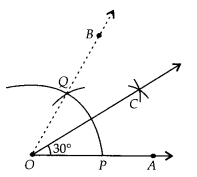(i) Draw $$\overline{O A}$$.
(ii) Taking O as centre and convenient radius, draw an arc, which intersects $$\overline{O A}$$ at P.
(iii) Taking P as centre and same radius, draw an arc which cut the previous arc at Q.
(iv) Join OQ and produce it to B. Thus, ∠BOA is the angle of 60°.
(v) Taking P as centre and radius more than half of PQ, draw an arc.
(vi) Taking Q as centre and with same radius, draw an arc which cut the previous arc at C.
(vii) Join OC. Thus ∠COA is the required angle of 30°.

(c) 90°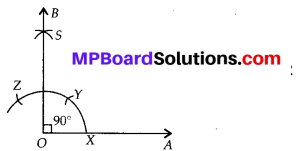(i) Draw $$\overline{O A}$$.
(ii) Taking O as centre and convenient radius, draw an arc, which intersects $$\overline{O A}$$ at X.
(iii) Taking X as centre and same radius, draw an arc which cut the previous arc at Y.
(iv) Taking Y as centre and same radius, draw another arc intersecting the arc drawn in step (ii) at ∠.
(v) Taking Y and ∠ as centres and same radius, draw two arcs intersecting each other at S.
(vi) Join OS and produce it to form a ray OB. Thus, ∠BOA is required angle of 90°.

(d) 120°(i) Draw $$\overline{O A}$$.
(ii) Taking O as centre and convenient radius, draw an arc, which intersects $$\overline{O A}$$ at P.
(iii) Taking P as centre and same radius, draw an arc which cut the previous arc at Q.
(iv) Taking Q as centre and same radius draw another arc intersecting the arc drawn in step (ii) at S.
(v) Join OS and produce it to D.
Thus, ∠AOD is the required angle of 120°.

(e) 45°(i) Draw $$\overline{O A}$$.
(ii) Taking O as centre and convenient radius, draw an arc, which intersects $$\overline{O A}$$ at X. .
(iii) Taking X as centre and same radius, draw an arc which cut the previous arc at Y.
(iv) Taking Y as centre and same radius, draw another arc intersecting the arc drawn in step (ii) at Z.
(v) Taking Y and Z as centres and same radius, draw two arcs intersecting each other at S.
(vi) Join OS and produce it to B. Thus, ∠BOA is the angle of 90°.
(vii) Draw OM the bisector of ∠BOA.
Thus, ∠MOA is the required angle of 45°.

(f) 135°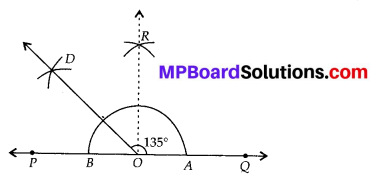(i) Draw $$\overline{P Q}$$ and take a point O on it.
(ii) Taking O as centre and convenient radius, draw an arc, which intersects $$\overline{P Q}$$ at A and B.
(iii) Taking A and B as centres and radius more than half of AB, draw two arcs intersecting each other at R.
(iv) Join OR. Thus, ∠QOR = ∠POR = 90°.
(v) Draw $$\overline{O D}$$ the bisector of ∠POR.
Thus, ∠QOD is the required angle of 135°.

Question 6.
Draw an angle of measure 45° and bisect it.
Solution:
Steps of construction :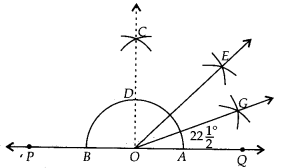(i) Draw a line PQ and take a point O on it.
(ii) Taking O as centre and a convenient radius, draw an arc which intersects $$\overline{P Q}$$ at two points A and B.
(iii) Taking A and B as centres and radius more than half of AB, draw two arcs which intersect each other at C.
(iv) Join OC. Then ∠COQ is an angle of 90°.
(v) Draw $$\overline{O E}$$ as the bisector of ∠COQ. Thus ∠QOE = 45°.
(vi) Again draw $$\overline{O G}$$ as the bisector of ∠QOE.
Thus, ∠QOG = ∠EOG = $$22 \frac{1}{2}^{\circ}$$.Question 7.
Draw an angle of measure 135° and bisect it.
Solution:
Steps of construction :(i) Draw a line $$\overline{P Q}$$ and take a point O on it.
(ii) Taking O as centre and convenient radius, draw an arc, which intersects $$\overline{P Q}$$ at A and B.
(iii) Taking A. and B as centres and radius more than half of AB, draw two arcs intersecting each other at R.
(iv) Join OR. Thus, ∠QOR = ∠POR = 90°.
(v) Draw $$\overline{O D}$$ the bisector of ∠POR. Thus, ∠QOD is the required angle of 135°.
(vi) Now, draw $$\overline{O E}$$ as the bisector of ∠QOD.
Thus, ∠QOE = ∠DOE = $$67 \frac{1}{2}^{\circ}$$ .

Question 8.
Draw an angle of 70°. Make a copy of it using only a straight edge and compasses.
Solution:
Steps of construction:(i) Draw an angle of 70° with the help of protractor, i.e., ∠POQ = 70°.
(ii) Draw $$\overline{A B}$$.
(iii) Place the compasses at O and draw an arc to cut the rays of ∠POQ at L and M.
(iv) Use the same compasses setting to draw an arc with A as centre, cutting AB at X.
(v) Set your compasses setting to the length LM with the same radius.
(vi) Place the compasses pointer at X and draw the arc to cut the arc drawn earlier at Y.
(vii) Join AY.
Thus, ∠YAX = 70°.

Question 9.
Draw an angle of 40°. Copy its supplementary angle.
Solution:
Steps of construction :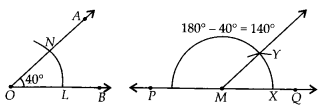(i) Draw an angle of 40° with the help of protractor, i.e., ∠AOB = 40°.
(ii) Draw a line $$\overline{P Q}$$.
(iii) Take any point M on PQ.
(iv) Place the compasses at O and draw an arc to cut the rays of ∠AOB at L and N.
(v) Use the same compasses setting to draw an arc M as centre, cutting MQ at X.